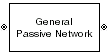# General Passive Network

Model two-port passive network described by `rfdata` object

• Library:
• RF Blockset / Equivalent Baseband / Black Box Elements

•## Description

The General Passive Network block models the two-port passive network described by an RF Toolbox™ data (`rfdata.data`) object.

If network parameter data and their corresponding frequencies exist as S-parameters in the `rfdata.data` object, the General Passive Network block interpolates the S-parameters to determine their values at the modeling frequencies. If the block contains network Y- or Z-parameters, the block first converts them to S-parameters. See Map Network Parameters to Modeling Frequencies for more details.

To learn how you can use General Passive Network block to model 2-port bandpass filter, see Import Data Files into RF Blocks section of Specify or Import Component Data example.

## Parameters

expand all

### Main

Data source that describes the passive device behaviour, specified as a `Data file` or an `RFDATA` object. The data source must contain network parameters and may also include noise data, nonlinearity data, or both.

Name of file that contains the passive device data, specified as a string or a character vector. The file name must include the extension. If the file is not in your MATLAB® path, specify the full path to the file or click the button to find the file.

#### Dependencies

To enable this parameter, choose ```Data file``` in Data source.

RF data object that contains passive device data, specified as an RF Toolbox `rfdata.data` object. You can also use an expression such as the default value to create an `rfdata.data` object from an `.s2p` file.

Method to interpolate the network parameters, specified as one of the following:

MethodDescription
`Linear`Linear interpolation
`Spline`Cubic spline interpolation
`Cubic`Piecewise cubic Hermite interpolation

### Visualization

Frequency data source, specified as ```Extracted from data source``` or `User-specified`.

Frequency data range, specified as a vector with each element unit in hertz.

Reference impedance, specified as a nonnegative scalar in ohms.

Type of data plot to visualize using the given data, specified as one of the following:

• `X-Y plane` — Generate a Cartesian plot of the data versus frequency. To create linear, semilog, or log-log plots, set the Y-axis scale and X-axis scale accordingly.

• `Composite data` — Plot the composite data.

• `Polar plane` — Generate a polar plot of the data. The block plots only the range of data corresponding to the specified frequencies.

• `Z smith chart`, ```Y smith chart```, and `ZY smith chart` — Generate a Smith® chart. The block plots only the range of data corresponding to the specified frequencies.

Type of S-Parameters to plot, specified as one of the following. When noise is spectral, `NF` plotting is possible.

 `S11` `S12` `S21` `S22` `GroupDelay` `GammaIn` `GammaOut` `VSWRIn` `VSWROut` `OIP3` `IIP3` `NF` `NFactor` `NTemp` `TF1` `TF2` `TF3` `Gt` `Ga` `Gp` `Gmag` `Gmsg` `GammaMS` `GammaML` `K` `Delta` `Mu` `MuPrime`

Type of S-Parameters to plot, specified as one of the following. When noise is spectral, `NF` plotting is possible.

 `S11` `S12` `S21` `S22` `GroupDelay` `GammaIn` `GammaOut` `VSWRIn` `VSWROut` `OIP3` `IIP3` `NF` `NFactor` `NTemp` `TF1` `TF2` `TF3` `Gt` `Ga` `Gp` `Gmag` `Gmsg` `GammaMS` `GammaML` `K` `Delta` `Mu` `MuPrime`

Plot format, specified as one of the following.

 `dB` `Magnitude (decibels)` `Abs` `Mag` `Magnitude (linear)` `Angle` `Angle(degrees)` `Angle(radians)` `Real` `Imag` `Imaginary`

Plot format, specified as one of the following.

 `dB` `Magnitude (decibels)` `Abs` `Mag` `Magnitude (linear)` `Angle` `Angle(degrees)` `Angle(radians)` `Real` `Imag` `Imaginary`

Frequency plot, specified as `Freq`.

Frequency plot format, specified as one of the following.

 `Auto` `Hz` `kHz` `MHz` `GHz` `THz`

Y-axis scale, specified as `Linear` or `Log`.

X-axis scale, specified as `Linear` or `Log`.

Plot specified data using the plot button.